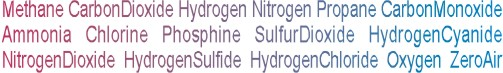Calibration gas blends, mixes and accessories
NON-REACTIVE SINGLE GAS > ZERO AIR :    5 Items found.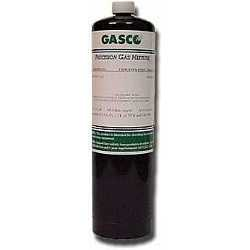(994)

NORLAB EQUIVALENT  P1002

Norlab Equivalent Calibration Gas Zero Air (20.9% Oxygen) Balance nitrogen 17L Liter cylinder (Made by Gasco) Ultra zero, less than 0.5ppm THC

Configure

\$36.47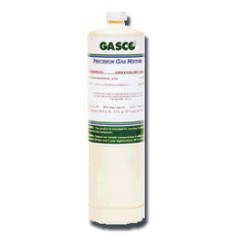(995)

NORLAB Equivalent  HP1002

Norlab Equivalent Calibration Gas Zero Air (20.9% Oxygen) Balance nitrogen 34LS Liter cylinder (Made by Gasco) Ultra zero, less than 0.5ppm THC

Configure

\$51.53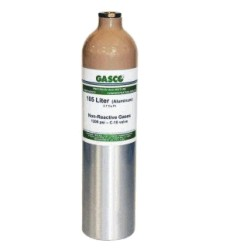(996)

NORLAB EQUIVALENT  1002

Norlab Equivalent Calibration Gas Zero Air (20.9% Oxygen) Balance nitrogen 105L Liter cylinder (Made by Gasco) Ultra zero, less than 0.5ppm THC

Configure

\$109.41(997)

NORLAB EQUIVALENT  IM1002

Norlab Equivalent Calibration Gas Zero Air (20.9% Oxygen) Balance nitrogen 221L Liter cylinder (Made by Gasco) Ultra zero, less than 0.5ppm THC

Configure

\$118.06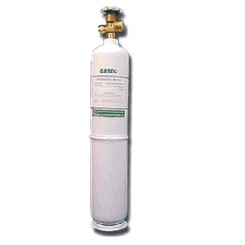(998)

NORLAB Equivalent  E1002

Norlab Equivalent Calibration Gas Zero Air (20.9% Oxygen) Balance nitrogen 552L Liter cylinder (Made by Gasco) Ultra zero, less than 0.5ppm THC

\$224.41